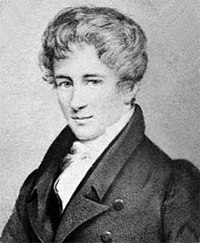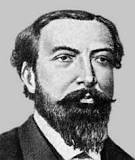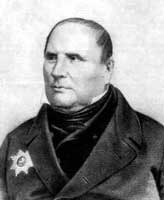# Preface

This section gives an introduction to the fundamental sets of solutions and the main tool---the Wronskian.
Return to computing page for the first course APMA0330
Return to computing page for the second course APMA0340
Return to Mathematica tutorial for the first course APMA0330
Return to Mathematica tutorial for the second course APMA0340
Return to the main page for the course APMA0330
Return to the main page for the course APMA0340
Return to Part IV of the course APMA0330

# Fundamental Sets of Solutions

A set of m functions $$\left\{ f_1 (x), \ f_2 (x), \ \ldots , \ f_m (x) \right\} ,$$ each defined and continuous on some interval $$|a, b| , \quad a < b,$$ is said to be linearly dependent on this interval if there exist constants $$k_1 , k_2 , \ldots , k_m$$ not all of them zero, such that

$k_1 f_1 (x) + k_2 f_2 (x) + \cdots + k_m f_m (x) \equiv 0 , \qquad x \in |a,b| ,$
for every x in the interval |𝑎,b|. Otherwise, the functions $$\left\{ f_1 (x), \ f_2 (x), \ \ldots , \ f_m (x) \right\} ,$$ are said to be linearly independent.
Two functions f and g are linearly dependent if and only if one of them is a constant multiple of another.

A set of functions $$\left\{ f_1 , \ f_2 , \ \ldots , \ f_m \right\}$$ is linearly dependent on an interval if and only if at least one of these functions can be expressed as a linear combination of the remaining functions (with at least one nonzero coefficient). The interval on which functions are defined plays a crucial role in this definition: the set of functions can be linearly independent on some interval, but can become dependent on another one (see the following example).

Example: This seems to have been pointed out for the first time by G. Peano, who considered two functions $$f(x) =x^2 \quad \mbox{and} \quad g(x) = x|x| .$$ These two functions are linearly dependent on any interval not containing zero, but they become linearly independent on any interval containing zero.     ■

Let

\begin{equation} \label{EqFund.1} L \left[ x, \texttt{D} \right] = a_n \texttt{D}^n + a_{n-1} \texttt{D}^{n-1} + \cdots + a_1 \texttt{D}^1 + a_0 \texttt{I} , \qquad a_n \ne 0, \end{equation}
where $$\texttt{D} = {\text d}/{\text d}x$$ is the derivative operator and $$\texttt{D}^0 = \texttt{I}$$ is the identity operator, be the linear differential operator of order n.
Any set $$\left\{ y_1 (x), \ y_2 (x), \ \ldots , \ y_n (x) \right\}$$ of n linearly independent solutions of the homogeneous linear n-th order differential equation $$L\left[ x,\texttt{D} \right] y =0$$ on an interval |𝑎,b| is said to be a fundamental set of solutions on this interval.
Theorem 1: There exists a fundamental set of solutions for the homogeneous linear n-th order differential equation $$L\left[ x,\texttt{D} \right] y =0$$ with continuous coefficients on an interval |𝑎,b|.

History of J.Wronski:

Jozéf Maria Hoëné Wronski was named Josef Hoëné after his birth in the town of Wolsztyn about 60 km south west of Poznań. His parents, from Czech families that had settled in western Poland, were Elzbieta Pernicka and Antoni Hoehne who was the municipal architect of Poznań. Josef Hoëné was educated in Poznań and Warsaw, but in 1794 he became involved in the Kosciuszko Uprising.

Despite battles for independence, Russia and Prussia partitioned Poland in 1793. General Tadeusz Kosciuszko, a hero of the American War of Independence, returned to Poland in 1794 and gathered an army of Poles to again fight for independence. Hoëné joined Kosciuszko as second lieutenant in the artillery. After winning the battle of Raclawice and capturing Warsaw and Wilno, Kosciuszko's army was defeated by Russian and Prussian forces after a siege of Warsaw. Kosciuszko was wounded and taken prisoner by the Russian army. Hoëné Wronski was also taken prisoner, and made to serve in the Russian army which he did until 1797 when he was released having reached the rank of lieutenant colonel.

He spent the next years in Germany studying philosophy at a number of different universities. He enlisted in the Polish Legion at Marseilles, in France, and become a French citizen in 1800. Then in 1810 he moved to Paris and, in the same year, he married Victoire Henriette Sarrazin de Mountferrier, whose brother was the mathematician Alexandre Mountferrier. It was at this time that he adopted the surname Wronski but he did not use it consistently, rather alternately used Wronski and Hoehne.

His main work involved applying philosophy to mathematics, the philosophy taking precedence over rigorous mathematical proofs. He wrote on the philosophy of mathematics. His book Introduction to a course in mathematics was published in London in 1821. He criticized Lagrange's use of infinite series and introduced his own ideas for series expansions of a function. He introduced his Wronski series, whose coefficients are determinants now known as Wronskians. The term "Wronskian" was coined by the Scottish mathematician Thomas Muir (1844--1934) in 1882. However, Wronskian is a particular case of more general determinant known as Lagutinski determinant (Mikhail Nikolaevich Lagutinski (1871–1915) was a Russian mathematician).

Let $$\left\{ f_1 , \ f_2 , \ \ldots , \ f_m \right\}$$ be m functions that together with their first m-1 derivatives are continuous on an interval |a,b|. The Wronskian or the Wronskian determinant of $$\left\{ f_1 , \ f_2 , \ \ldots , \ f_m \right\}$$ evaluated at $$x \in |a, b|$$ is denoted by $$W[f_1, f_2 , \ldots , f_m ](x)$$ or $$W(f_1, f_2 , \ldots , f_m ; x )$$ or simply $$W(x)$$ and is defined to be the determinant
\begin{equation} \label{EqFund.2} W[f_1, f_2 , \ldots , f_m ](x) = \det \begin{bmatrix} f_1 & f_2 & \cdots & f_m \\ f'_1 & f'_2 & \cdots & f'_m \\ f''_1 & f''_2 & \cdots & f''_m \\ \vdots & \vdots & \ddots & \vdots \\ f_1^{(m-1)} & f_2^{(m-1)} & \cdots & f_m^{(m-1)} \end{bmatrix} . \end{equation}
Each of the functions appearing in this determinant is to be evaluated at $$x \in |a, b| .$$

For the special case m = 2, the Wronskian takes the form

$W[ f_1 , f_2 ] (x) = \begin{vmatrix} f_1 (x) & f_2 (x) \\ f'_1 (x) & f'_2 (x) \end{vmatrix} = f_1 (x)\, f'_2 (x) - f'_1 (x)\, f_2 (x) .$
Lemma: For arbitrary functions $$\left\{ f , \ g_1 , \ \ldots , \ g_{n-1} \right\} ,$$ the Wronskian determinants satisfy the equation
\begin{equation} \label{EqFund.3} \det \left[ \frac{{\text d}^j \left( f \,g_k \right)}{{\text d} x^j} \right]_{j,k =0,1,\ldots , n-1} = f^n \, \det \left[ \frac{{\text d}^j \,g_k}{{\text d} x^j} \right]_{j,k =0,1,\ldots , n-1} . \end{equation}
In particular,
\begin{align*} W[ f, f\,g ] (x) &= \det \begin{bmatrix} f & f\,g \\ f' & f'\,g + f\, g' \end{bmatrix} = f^2 \begin{vmatrix} 1 & g \\ 0 & g' \end{vmatrix} = f^2 \, g' = f^2 \, W[1, g] , \\ W[ f, f\,g_1 , f\, g_2 ] (x) &= \begin{vmatrix} f & f\,g_1 & f\, g_2 \\ f' & \left( f\,g_1 \right)' & \left( f\,g_2 \right)' \\ f'' & \left( f\,g_1 \right)'' & \left( f\,g_2 \right)'' \end{vmatrix} = f^3 \,W[1, g_1 , g_2 ] . \end{align*}
Theorem 2: Let $$\left\{ f_1 , \ f_2 , \ \ldots , \ f_{m} \right\}$$ be m functions that together with their first m-1 derivatives are continuous on an open interval (𝑎,b). If their Wronskian $$W \left[ f_1 , f_2 , \ldots , f_{m} \right] (x_0 )$$ is not equal to zero at some point $$x_0 \in (a,b) ,$$ then these functions $$f_1 , \ f_2 , \ \ldots , \ f_{m}$$ are linearly independent on (a,b). Alternatively, if $$\left\{ f_1 , \ f_2 , \ \ldots , \ f_{m} \right\}$$ are linearly dependent and they have m-1 first derivatives on the open interval (𝑎,b), then their Wronskian $$W \left[ f_1 , f_2 , \ldots , f_{m} \right] (x ) \equiv 0$$ for every x in (𝑎,b).
Theorem 3: A finite set of linearly independent holomorphic (represented by convergent power series) functions has a nonzero Wronskian.
Theorem 4: Let $$\left\{ y_1 , \ y_2 , \ \ldots , \ y_{n} \right\}$$ be the solutions of the n-th order linear differential equation
\begin{equation} \label{EqFund.4} y^{(n)} + a_{n-1} (x)\, y^{(n-1)} + \cdots + a_1 (x)\, y' + a_0 (x)\, y =0 \end{equation}
with continuous coefficients $$a_0 (x), \ a_1 (x) , \ \ldots , \ a_{n-1} (x) ,$$ defined on an open interval (a,b). Then functions $$\left\{ y_1 , \ y_2 , \ \ldots , \ y_{n} \right\}$$ are linearly independent on this interval (𝑎,b) if and only if their Wronskian never vanishes on (𝑎,b). Alternatively, these functions $$\left\{ y_1 , \ y_2 , \ \ldots , \ y_{n} \right\}$$ are linearly dependent on this interval (𝑎,b) if and only if $$W \left[ y_1 , y_2 , \ldots , y_{n} \right] (x ) \equiv 0$$ is zero for all x ∈ (𝑎,b).
Theorem 5: If y = ϕ1 and y = ϕ2 are solutions of the second order linear differential equation
\begin{equation} \label{EqFund.5} y'' + p (x)\, y' + q (x)\, y =0, \end{equation}
where p(x) and q(x) are continuous on an open interval (𝑎,b), then the Wronskian $$W(x) = W[ \phi_1 , \phi_2 ] (x)$$ is given by
\begin{equation} \label{EqFund.6} W[ \phi_1 , \phi_2 ] (x) = W[ \phi_1 , \phi_2 ] (x_0 ) \, \exp \left\{ - \int_{x_0}^x p(t)\,{\text d} t \right\} . \end{equation}
Here x0 is any point from the interval (𝑎,b).
To prove Abel's theorem, we start by noting that ϕ1 and ϕ2 satisfy
$\begin{split} \phi''_1 + p(x)\,\phi'_1 + q(x)\,\phi_1 &= 0, \\ \phi''_2 + p(x)\,\phi'_2 + q(x)\,\phi_2 &= 0. \end{split}$
If we multiply the first equation by -ϕ2, multiply the second by ϕ1, and add the resulting equations, we obtain
$\left( \phi_1 \phi''_2 - \phi''_1 \phi_2 \right) + p(x) \left( \phi_1 \phi'_2 - \phi'_1 \phi_2 \right) = 0 .$
Let denote by W(x) the Wronskian of solutions ϕ1 and ϕ2, and observe that
$W' = \phi_1 \phi''_2 - \phi''_1 \phi_2 .$
This allows us to write
$\left( \phi_1 \phi''_2 - \phi''_1 \phi_2 \right) + p(x) \left( \phi_1 \phi'_2 - \phi'_1 \phi_2 \right) = W' + p(x)\, W = 0 .$
The equation for W can be solves immediately since it is both a first order linear homogeneous equation and a separable equation. This complete the proof.    ▣The formula above was derived by the greatest Norwegian mathematician Niels Henrik Abel (1802--1829) in 1827 for second order differential equation. In general case, namely, for the equation $$y^{(n)} + a_{n-1} (x)\, y^{(n-1)} + \cdots + a_1 (x)\, y' + a_0 (x)\, y =0 ,$$ the French mathematician Joseph Liouville (1809--1882) and the Russian mathematician Michel Ostrogradski (1801--1861) independently proved in 1838 the following theorem.

Theorem Liouville--Ostrogradski: Assume that ϕ1(x), ϕ2(x), … , ϕn(x) are n linearly independent solutions of the homogeneous differential equation $$y^{(n)} + a_{n-1} (x)\, y^{(n-1)} + \cdots + a_1 (x)\, y' + a_0 (x)\, y =0 ,$$ and let Wn(x) be the Wronsian of this functions. Then Wn(x) is a solution of the differential equation
$\frac{{\text d}W_n}{{\text d}x} + a_{n-1} W_n (x) = 0 \qquad \Longleftrightarrow \qquad W_n (x) = W\left( x_0 \right) \exp \left\{ - \int_{x_0}^x a_{n-1} (t)\,{\text d} t \right\} .$
A determinant is differentiated by differentiating each term in the i-th row and then summing all the n resulting determinants for i = 1, 2, … , n. By differentiating the i-th row in Wn for in - 1, we obtain row number (i+1), so the contribution becomes zero for in - 1. Hence,
$\frac{{\text d}W_n}{{\text d}x} = \begin{vmatrix} \phi_1 & \phi_2 & \cdots & \phi_n \\ \vdots & \vdots & \ddots & \vdots \\ \phi_1^{(n-2)} & \phi_2^{(n-2)} & \cdots & \phi_n^{(n-2)} \\ \phi_1^{(n)} & \phi_2^{(n)} & \cdots & \phi_n^{(n)} \end{vmatrix} .$
Then the claimed differential equation for the Wronskian becomes
$\frac{{\text d}W_n}{{\text d}x} + a_{n-1} W_n = \begin{vmatrix} \phi_1 & \phi_2 & \cdots & \phi_n \\ \vdots & \vdots & \ddots & \vdots \\ \phi_1^{(n-2)} & \phi_2^{(n-2)} & \cdots & \phi_n^{(n-2)} \\ \phi_1^{(n)} + a_{n-1} \phi_1^{(n-1)} & \phi_2^{(n)} + a_{n-1} \phi_2^{(n-1)}& \cdots & \phi_n^{(n)} + a_{n-1} \phi_n^{(n-1)} \end{vmatrix} = 0,$
where we just add 𝑎n-1 times the i-th row for i = 1, 2, … , n-1 to the last row and use that ϕ1(x), ϕ2(x), … , ϕn(x) are solutions of the homogeneous differential equation.    ▣
Remark: Repeating the preceding proof with suitable modifications, we more generally obtain that each Wronskian Wk of first k linearly independent solutions of the given linear differential equation of order n satisfies the differential equation
$\frac{{\text d}^{n+1-k} W_k}{{\text d}x^{n+1-k}} + a_{n-1} \frac{{\text d}^{n-k} W_k}{{\text d}x^{n-k}} + \cdots + a_{k-1} (x)\,W_k = 0, \qquad k=1,2,\ldots , n .$
Here Wk(x) for n functions $$\varphi_1 , \varphi_2 , \ldots , \varphi_n$$ is
$W_k \left[ \varphi_1 , \ldots , \varphi_k \right] (x) = \det \begin{bmatrix} \varphi_1 & \varphi_2 & \cdots & \varphi_k \\ \varphi'_1 & \varphi'_2 & \cdots & \varphi'_k \\ \vdots & \vdots & \ddots & \vdots \\ \varphi_1^{(k-1)} & \varphi_2^{(k-1)} & \cdots & \varphi_k^{(k-1)} \end{bmatrix} , \qquad k=1,2,\ldots , n.$
Corollary: If the Wronskian W(x) of solutions $$y^{(n)} + a_{n-1} (x)\, y^{(n-1)} + \cdots + a_1 (x)\, y' + a_0 (x)\, y =0 ,$$ is zero at one point x0 of an interval (𝑎,b) where coefficients are continuous, then W(x) is 0 for all $$x \in (a,b) .$$
A Wronskian is a constant if and only if the coefficient 𝑎n-1 = 0.
Corollary: If the Wronskian W(x) of solutions $$y^{(n)} + a_{n-1} (x)\, y^{(n-1)} + \cdots + a_1 (x)\, y' + a_0 (x)\, y =0 ,$$ is not zero, then it is not zero at every point of an interval where coefficients are continuous.
Theorem 7: There exists a fundamental set of solutions for the homogeneous linear n-th order linear differential equation in an interval where all coefficients are continuous.
Theorem: Consider the initial value problem
$y'' + p(x)\, y' + q(x)\, y =0, \qquad y(x_0 ) = y_0 , \quad y' (x_0 ) = y_1 ,$
where p(x) and q(x) are continuous on an open interval (𝑎,b) that contains the point x0. Suppose that y1 and y2 are two linearly independent solutions of the homogeneous equation $$y'' + p(x)\, y' + q(x)\, y =0 .$$ Then it is always possible to choose the constants C1 and C2 so that
$y = C_1 y_1 (x) + C_2 y_2 (x)$
is the unique solution to the given initial value problem.
Theorem: Consider the linear differential equation
$y'' + p(x)\, y' + q(x)\, y =0 ,$
whose coefficients p and q are continuous on some open interval (𝑎,b). Choose some point x0 in it. Let y1 be the solution of the above homogeneous linear equation that also satisfies the initial conditions
$y(x_0 ) =1, \qquad y' (x_0 ) =0,$
and let y2 be the solution of the above homogeneous linear equation that also satisfies the initial conditions
$y(x_0 ) = 0, \qquad y' (x_0 ) =1.$
Then y1 and y2 form a fundamental set of solutions to the equation $$y'' + p(x)\, y' + q(x)\, y =0 .$$

To check linearly independence of two functions, we have two options. First, two functions are linearly independent if and only if one of them is a constant multiple of another. Another option is to calculate the Wronskian if you know that these two functions are solutions of the same differential equation.

Example: Given two functions $$\{ x , 3x \} ,$$ determine whether they are linearly dependent. The Mathematica command Wronskian[{y1 ,y2, ...},x] gives the Wronskian determinant for the functions $$y1 (x), y2 (x) , \ldots ,$$ depending on x.

y={x,3 x}
w={y,D[y,x]}
Det[w]
Out= 0

Example: Consider two sets of functions

$A = \left\{ \sin x , x\,\sin x , x^2 \sin x \right\} \qquad \mbox{and} \qquad B= \left\{ 1 , \sin x , \sin x \right\} .$
Every element in the set A is multiple of elements from B by a single function---sine. So we verify the formula using Mathematica:
Wronskian[{1, x, x^2}, x]
2
Wronskian[{Sin[x], x*Sin[x], x^2*Sin[x]}, x]
2 Sin[x]^3
1. Boston, A. and Dumas, F., Wronskians and linear independence, The American Mathematical Monthly, 2010, Vol. 117, No. 8, pp. 722--727.
2. Malykh, M.D., M.N. Lagutinski method for integration of ordinary differential equations, 2016.